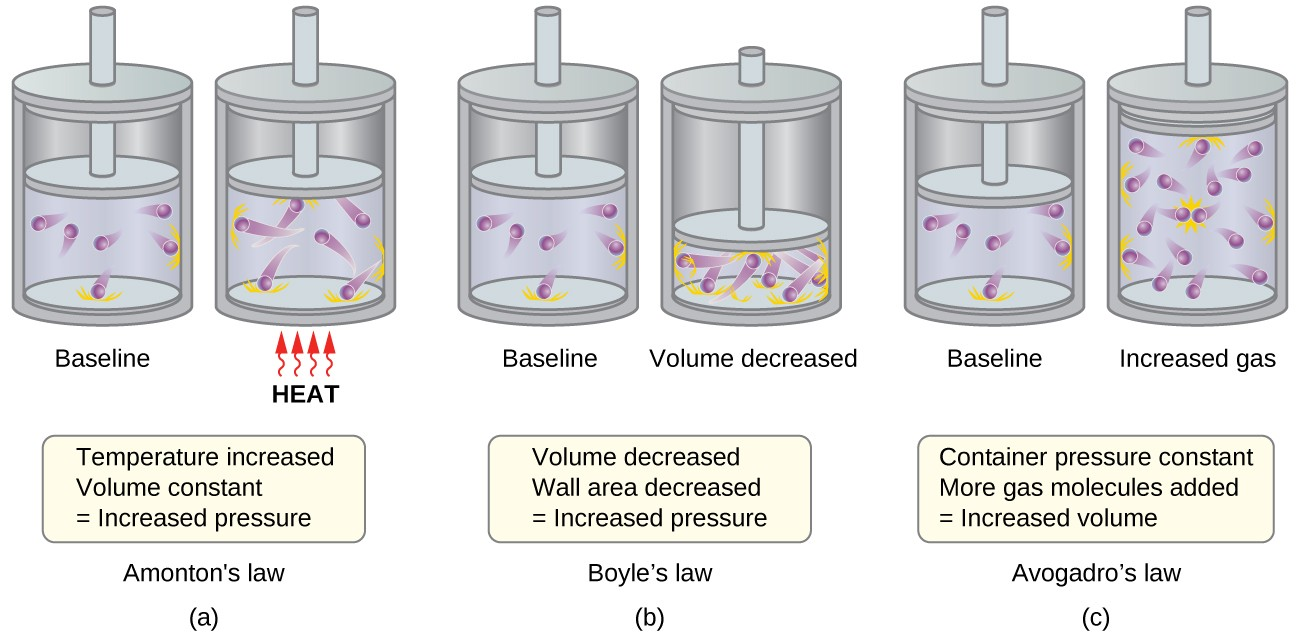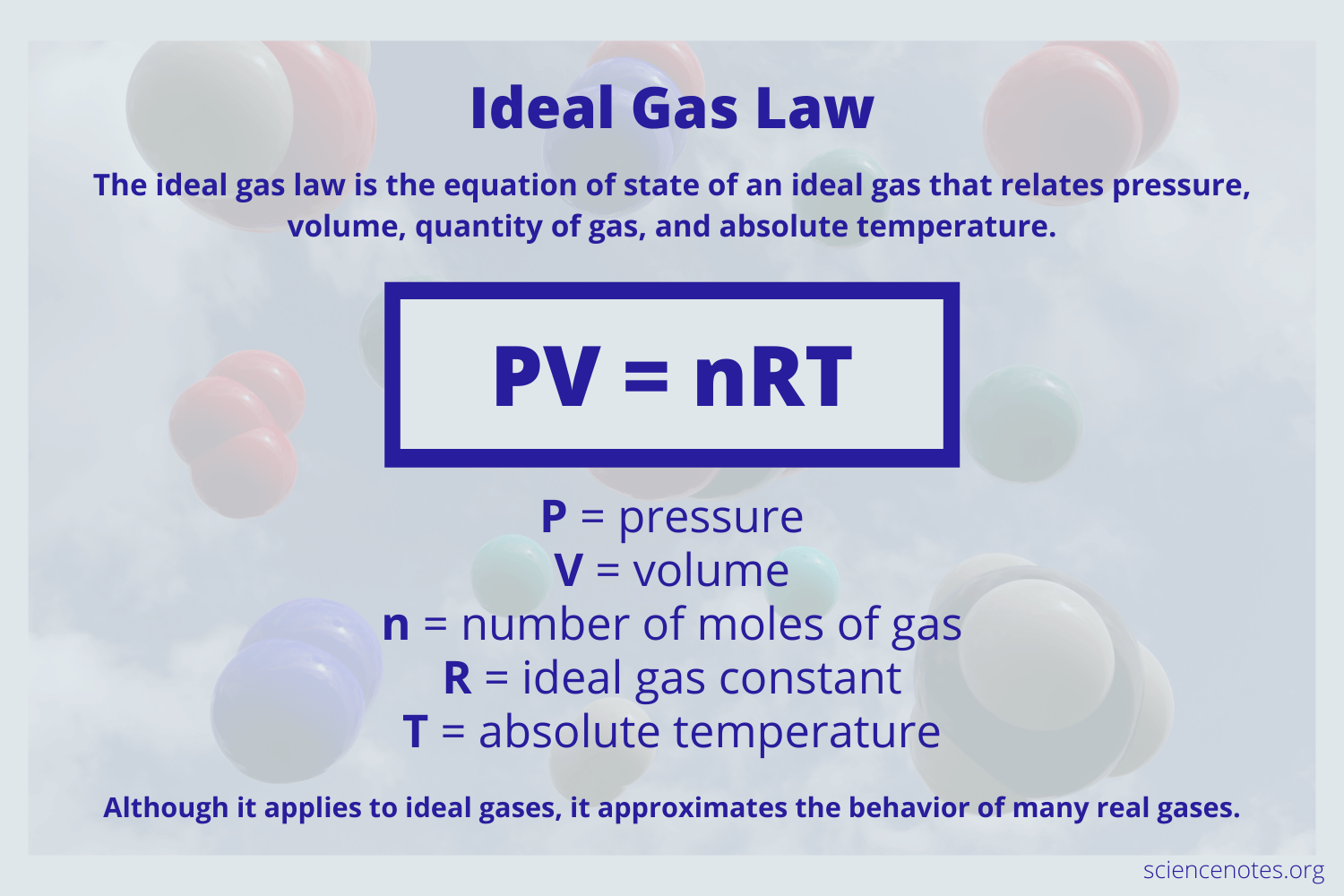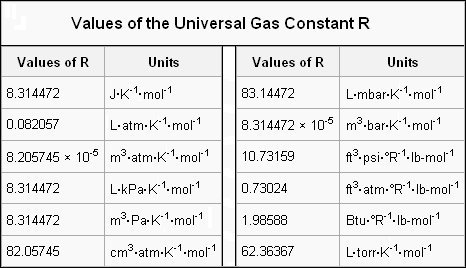# Ideal Gas Law Study Guide

Lesson Objectives

• Define the ideal gas law
• Describe the relationship between the amount of gas (n) volume (V), pressure (P), and temperature (T) as they relate to an ideal gas
• Solve problems using ideal gas law equation
• Derive the ideal gas law from Charles’s, Boyle’s, and Avogadro’s laws

## IntroductionSource

With the right information and equations, solids and liquids are fairly simple to understand when it comes to behavior. Gasses, however, are a bit more complicated. Gasses are composed of billions and billions of particles that collide and interact at an incredibly high speed. Because it’s difficult to characterize a real gas precisely, the notion of an ideal gas was developed as a rough approximation to simulate and forecast the behavior of real gasses. Let’s talk about what an ideal gas is, its characteristics, and limitations.

### What’s an ideal gas?

The term “ideal gas” describes a hypothetical gas made up of molecules that are neither attracted to nor repelled by one another, occupy no space, and all collisions between them are elastic (a collision in which no net kinetic energy is lost).

In reality, there is no such thing as a truly ideal gas. Still, many real gasses act closely to an ideal gas under certain conditions. Gasses such as nitrogen, oxygen, hydrogen, and the noble gasses are capable of being treated as ideal within reasonable bounds. In general, gasses tend to behave as ideal gasses at very high temperatures and low pressures (circumstances wherein individual particles move very rapidly and are very far apart from each other, with almost no engagement).Source

### The Ideal Gas Law Definition, Formula, & Derivation

The ideal gas law states that the product of the pressure and volume of one gram molecule is equal to the product of the gas’s actual temperature and the universal gas constant. We express this mathematically as:

PV = nRT

Where:

• P = pressure
• V = volume
• n = the amount of gas in moles
• R = the ideal gas constant
• T = the absolute temperature

The ideal gas law is derived from Charles’s, Boyle’s, Gay-Lussac’s, and Avogadro’s laws combined. Let’s take a look at how it’s done.

In the combined gas law of Charles, Boyle, and Gay-Lussac, the temperature of a gas is directly proportional to its pressure and inversely proportional to its volume. When we add Avogadro’s law to the mix, we find that volume or pressure are directly proportional to the number of moles in a gas. We express this combination with the following equation:

(P1V1)/(T1n1) = (P2V2)/(T2n2)

Where:

• P = pressure
• V = volume
• n = the amount of gas in moles
• T = the absolute temperature

We are now able to substitute one side of this equation to give us the constant R as we’re able to in the other gas law equations, expressing this as:

(PV)/(Tn) = R

When we reorganize this equation, we get the standard arrangement of the ideal gas law, which is:

PV = nRT

Where:

• R = the ideal gas constantSource

It’s important to make sure we utilize the correct units when performing calculations for the ideal gas law. If you’re using the gas constant 8.31, you’ll need to enter the pressure in pascals, volume in meter cubes, and temperature in kelvin. If you wish to use any other unit, you could use the value of gas constant accordingly:Source

### Sample Problem: Ideal Gas Law

What is the volume occupied by 5.43 g of carbon gas at a pressure of 56.9 kPA and temperature of 21°C?

Step 1: Define the variables

P = 56.9 kPAT = 21°C = 294.15 KR = 8.314 J/K•molMass C2 = 5.43 gCarbon = 12.0107 g/molV = ?

The mass of C2 must be converted to moles in order to use the ideal gas law.

5.43 x (1 mol C2)/12.0107g = 0.452 mol C2

Step 2: Choose an equation

For the purpose of this calculation, let’s use the standard notation of the ideal gas law, PV = nRT.

Step 3: Calculate

PV = nRTV = nRT/PV = (0.452 mol)(8.314 J/K•mol)(294.15 K)/(56.9 kPA)V = 19.4 L C2

The volume occupied by 5.43 g of carbon gas at a pressure of 56.9 kPA and temperature of 21 is 19.4 L.

## 💡 Summary

• When the particles of a gas are so far apart that they would not generate any attractive forces on each other, the gas is said to be ideal.

• The ideal gas law asserts that a gas’s pressure, temperature, and volume are all connected.

### 🧐 FAQs:

1. What does the ideal gas state?

The ideal gas law asserts that a gas’s pressure, temperature, and volume are all connected.

2. Why is the ideal gas inaccurate?

The ideal gas solely exists when the surrounding conditions are optimum. The molecule size and intermolecular forces become significant to consider at high pressure and low temperature, and they are no longer insignificant; hence the ideal gas law is no longer valid.

3. Does ideal gas law apply to liquids?

Liquids are not subject to the ideal gas law.

4. How is ideal gas law used in everyday life?

If a researcher wishes to store 750 g of oxygen in a vessel with a pressure of 2 atm and a temperature of 150 degrees Fahrenheit, the ideal gas law can be used to calculate the capacity of the container needed.

We hope you enjoyed studying this lesson and learned something cool about Ideal Gas law! Join our Discord community to get any questions you may have answered and to engage with other students just like you! Don’t forget to download our app to experience our fun VR classrooms – we promise it makes studying much more fun! 😎

## Sources

1. What is the Ideal Gas Law?: https://www.khanacademy.org/science/physics/thermodynamics/temp-kinetic-theory-ideal-gas-law/a/what-is-the-ideal-gas-law accessed 27 Feb 2022
2. Ideal Gas Law: https://byjus.com/physics/ideal-gas-law-and-absolute-zero/ accessed 27 Feb 2022
3. The Ideal Gas Law: https://courses.lumenlearning.com/boundless-chemistry/chapter/the-ideal-gas-law/ accessed 27 Feb 2022.
]]>